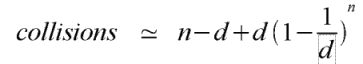# Advanced use of hash codes in Java: some brief statistics

To understand some advanced uses of hash maps that we're going to introduce, it's worth having a brief overview of some of the statistics involved.

### Collisions: chance of repeated hash codes

A collision is when two items end up with the same hash code, or with the same index in a hash table of a given size. If we take n hash codes from a possible range of d, then a rough formula for working out the number of expected collisions is as follows:(For a slightly more accurate calculation, you can also use the collision calculator opposite. This uses a more accurate formula discussed below.) The above approximation works well when d (the number of possible hash codes) is fairly large– say, at least in the hundreds– and n is much smaller than d (say, at most half, but ideally within an order of magnitude of the square root of d). In practice, this approximation can be stated in easier terms if you're dealing with hash codes and hash tables that are powers of two, as is often the case: Operating with x-bit hash codes, if we take 20.5x + 1 codes from this range, we'll expect 2 collisions. Doubling the number of items quadruples the number of collisions. Get the Hash Collision Calculator widget and many other great free widgets at Widgetbox!

For example, say we are using 32-bit hash codes (so x is 32). This is the normal range for hash codes of Java objects. This means that if we take the hash codes of 217 random objects, we would on average expect 2 collisions: that is, 2 different hash codes each shared by more than one object1. 217 is just over 130,000 objects. In other words:

• With 32-bit keys, the chance of having objects sharing the same hash code is fairly small, but needs to be considered when we start having a moderately large number of objects.

Even with a good hash function, if we put a few thousand objects into a hash table (or across a number of hash tables) then sooner or later, two objects will have the same hash code. So in practice for a general-purpose hash table such as Java's HashMap, the implementation has to store the keys to check that the mapping we're retrieving really is for the object sought. (Of course, these statistics assume a perfect hash function; in practice, things are a little worse.)

### Collisions in a hash table

Now we can generally apply the above formula in some cases to work out the number of actual collisions– that is, the number of buckets with more than one item– that we expect in a hash table of a given size. With typically-sized hash tables, the number of expected collisions becomes considerable. For example, if we store 1000 objects in a hash table of size 211 (2048), then we will expect 10002/4096 = over 200 collisions. Of course, upping the number of buckets in the hash table reduces the number of collisions: with 1000 objets in a hash table of size 216, we'd get fewer than 10 collisions (less than 1%). But usually, having a hash table so much bigger than the number of objects is extremely wasteful. So even if we store only the hash codes instead of the full keys in a hash table (a case we'll consider in a moment), we would still generally have to compare the hash code: bucket index alone isn't generally enough to confirm a match.

Where the number of items chosen approaches the hsah table size, the above approximation doesn't work so well (although in fact, it tends to overestimate the number of collisions). A better approximation, from which the above is derived2, is as follows:## Next...

On the next page, we exploit the above statistics to consider storing only the hash code in a hash map or hash set.

1. In all our calculations, we generally assume for the sake of argument that every collision involves a pair of objects. In reality, of course, it could be that more than 2 objects share the same hash code; our calculations would count this case as several collisions, when from one point of view it's still a single collision. This turns out not to matter a great deal: the calculations we give here are still a good guide in practice.
2. The derivation essentially consists of applying the binomial expansion to the term (1 - 1/d)n. The first three terms of the binomial expansion are 1 - n/d + n(n-1)/2d2 + .... Plugging these back into the formula gives the approximation n(n-1)/2d, which is of course close to n2/2d.